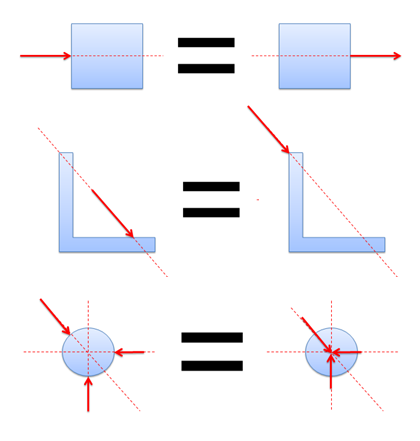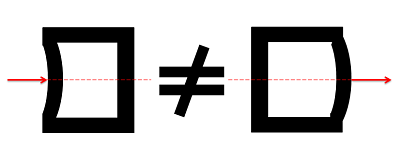﻿ Mechanics Map - Principle of Transmissibility

# Principle of Transmissibility

The principle of transmissibility states that the point of application of a force can be moved anywhere along its line of action without changing the external reaction forces on a rigid body. Any force that has the same magnitude and direction, and which has a point of application somewhere along the same line of action will cause the same acceleration and will result in the same moment. Therefore, the points of application of forces may be moved along the line of action to simplify the analysis of rigid bodies.Because of the principle of transmissibility, each of the above pairs is equivalent.

When analyzing the internal forces (stress) in a rigid body, the exact point of application does matter. This difference in stresses may also result in changes in geometry which will in turn affect reaction forces. For this reason, the principle of transmissibility should only be used when examining external forces on bodies that are assumed to be rigid.The exact point of application of a force will impact how internal forces (stresses) are distributed, so the principle of transmissibility cannot be applied when examining internal forces.